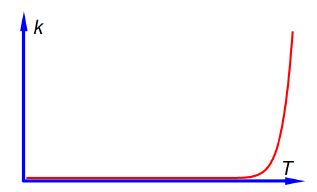Chemistry

# Explain Arrhenius Equation

Question 1:

The rate constant k of a chemical reaction increases with the absolute temperature T. This increase follows the equation below which is called the Arrhenius equation.

k = Ae-(Ea/RT)

Where Ea is the activation energy for the reaction, R is the gas constant and A is called the pre-exponential factor which is a constant for a given reaction. Sketch the shape of the graph of k versus T labelling the axes.

Question 2: The Arrhenius Equation

k = Ae-(Ea/RT)

Write the Arrhenius equation in the form of natural-logs.

Solution 1:

From the Arrhenius equation one can plot k against T as in below Figure.sketch of k against Tfor the Arrhenius equation.

In above Figure it is difficult to read as at high temperatures a small change in T gives drastic changes in the rate constant k but at low temperatures a large change in T gives a negligible change in k. It would be much better if we can rearrange the Arrhenius equation to enable us to have a linear (straight line) graph.

Solution 2:

We can go from the exponential form of the Arrhenius equation to the natural-log form by:

(1) taking natural logs on both sides,

(2) the log on the right is a product of two terms which can be separated as the sum of two logs,

(3) the second term on the right is a natural-log of an exponential which is just equal to the exponent.

ln (k) = ln [Ae-(Ea/RT)]

ln (k) = ln [A] + ln [e-(Ea/RT)]

ln (k) = [ln (A) – Ea/RT]